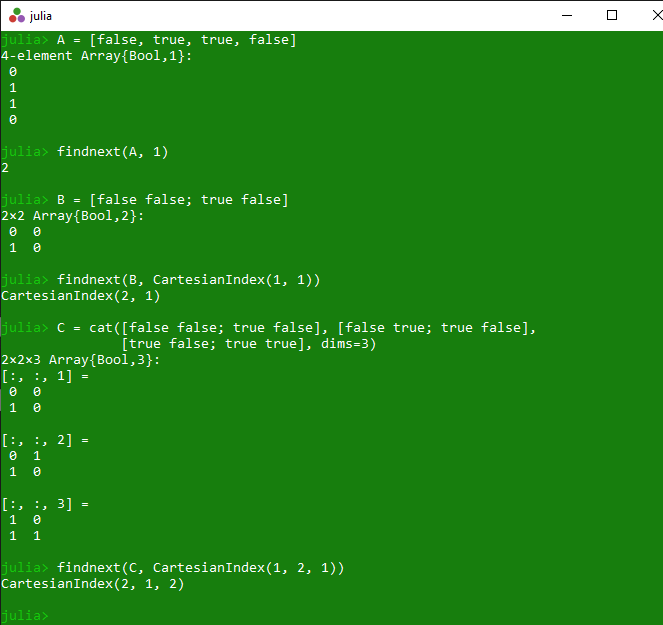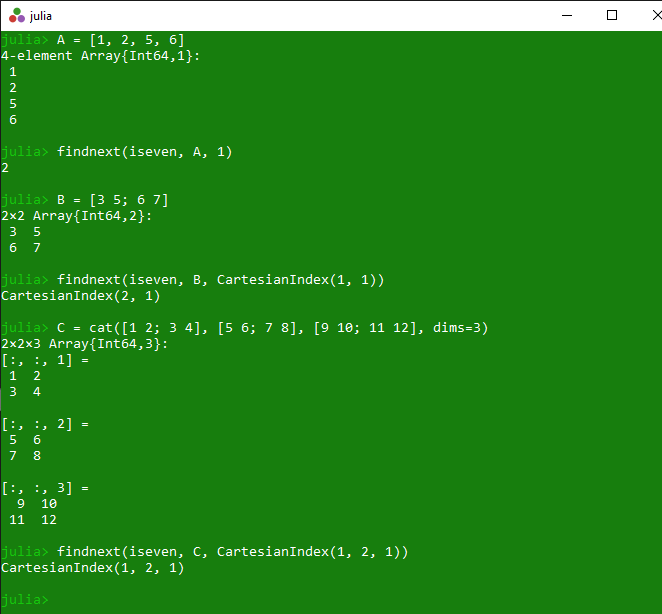Related Articles

# Get next true value from a given array index in Julia | Array findnext() Method

• Last Updated : 23 Mar, 2020

The `findnext()` is an inbuilt function in julia which is used to return the next coming index after or including i of a true element of the specified array A, or returns zero if true value is not found. Here values of index or key start from 1 i.e, for index of 1st element is 1, index of 2nd element is 2 and so on.

Syntax:
findnext(A, i)
or
findnext(predicate::Function, A, i)

Parameters:

• A: Specified array
• i: Specified value
• Predicate Function: Determines whether something is true or false based on the specified arguments

Returns: It returns the next coming index after or including i of a true element of the specified array A, or returns zero if true value is not found.

Example 1:

 `# Julia program to illustrate ``# the use of Array findnext() method`` ` `# Finding index of next true value coming ``# after or including index 1 from ``# the 1D array A``A ``=` `[false, true, true, false]``println(findnext(A, ``1``))`` ` `# Finding index of next true value coming ``# after or including index (1, 1) from``# the 2D array B of size 2 * 2``B ``=` `[false false; true false]``println(findnext(B, CartesianIndex(``1``, ``1``)))`` ` `# Finding index of next true value coming ``# after or including index (1, 2, 1) from``# the 3D array C of size 2 * 2*2``C ``=` `cat([false false; true false], ``        ``[false true; true false],``        ``[true false; true true], dims ``=` `3``)``println(findnext(C, CartesianIndex(``1``, ``2``, ``1``)))`

Output:Example 2:

 `# Julia program to illustrate ``# the use of Array findnext() method`` ` `# Finding index of next even value coming ``# after or including index 1 from ``# the 1D array A``A ``=` `[``1``, ``2``, ``5``, ``6``]``println(findnext(iseven, A, ``1``))`` ` `# Finding index of next even value coming ``# after or including index (1, 1) from ``# the 2D array B of size 2 * 2``B ``=` `[``3` `5``; ``6` `7``]``println(findnext(iseven, B, CartesianIndex(``1``, ``1``)))`` ` `# Finding index of next even value coming ``# after or including index (1, 2, 1) from ``# the 3D array C of size 2 * 2*2``C ``=` `cat([``1` `2``; ``3` `4``], [``5` `6``; ``7` `8``], ``        ``[``9` `10``; ``11` `12``], dims ``=` `3``)``println(findnext(iseven, C, CartesianIndex(``1``, ``2``, ``1``)))`

Output:My Personal Notes arrow_drop_up